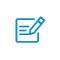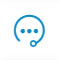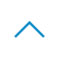# 如何在TensorFlow 2.0中构建强化学习智能体

TensorFlow 2.0 即将在今年上半年推出正式版，虽然目前有预览版可供使用，但是对于大多数人来说，新版本能够带来什么还没有具体的概念。本文将简要介绍在 TensorFlow 2.0 上使用强化学习算法的体验。其中作者概述了 Keras 子类 API、Eager Execution、会话替换以及会让开发更加方便的技巧。 可思数据sykv.com,sykv.cn

TensorFlow 2.0 版的宗旨是让开发者们能够更轻松，在深度强化学习上这一理念显然也得到了发扬：在这个例子中，我们的智能体源代码不到 150 行!如果你想看看代码，Python 文件格式的在这里：https://github.com/inoryy/tensorflow2-deep-reinforcement-learning 本文来自可思数据(sykv.com)，转载请联系本站及注明出处

> conda create -n tf2 python=3.6
> source activate tf2
> pip install tf-nightly-2.0-preview # tf-nightly-gpu-2.0-preview for GPU version 可思数据-数据挖掘,智慧医疗,机器视觉,机器人sykv.com

>>> import tensorflow as tf
>>> print(tf.__version__)1.13.0-dev20190117
>>> print(tf.executing_eagerly()) 可思数据sykv.com,sykv.cn

True 可思数据-人工智能资讯平台sykv.com

>>> print(tf.reduce_sum([1, 2, 3, 4, 5]))
tf.Tensor(15, shape=(), dtype=int32) 可思数据-AI,sykv.com智能驾驶,人脸识别,区块链,大数据

``````import numpy as npimport tensorflow as tfimport tensorflow.keras.layers as kl
class ProbabilityDistribution(tf.keras.Model):def call(self, logits):# sample a random categorical action from given logitsreturn tf.squeeze(tf.random.categorical(logits, 1), axis=-1)
class Model(tf.keras.Model):def __init__(self, num_actions):
super().__init__('mlp_policy')
# no tf.get_variable(), just simple Keras API
self.hidden1 = kl.Dense(128, activation='relu')
self.hidden2 = kl.Dense(128, activation='relu')
self.value = kl.Dense(1, name='value')
# logits are unnormalized log probabilities
self.logits = kl.Dense(num_actions, name='policy_logits')
self.dist = ProbabilityDistribution()

def call(self, inputs):# inputs is a numpy array, convert to Tensor
x = tf.convert_to_tensor(inputs, dtype=tf.float32)
# separate hidden layers from the same input tensor
hidden_logs = self.hidden1(x)
hidden_vals = self.hidden2(x)
return self.logits(hidden_logs), self.value(hidden_vals)

def action_value(self, obs):# executes call() under the hood
logits, value = self.predict(obs)
action = self.dist.predict(logits)
# a simpler option, will become clear later why we don't use it# action = tf.random.categorical(logits, 1)return np.squeeze(action, axis=-1), np.squeeze(value, axis=-1)``` 可思数据sykv.com,sykv.cn ```

``````import gym

env = gym.make('CartPole-v0')
model = Model(num_actions=env.action_space.n)

obs = env.reset()# no feed_dict or tf.Session() needed at all
action, value = model.action_value(obs[None, :])
print(action, value) #  [-0.00145713]``` 可思数据sykv.com ```

``````class A2CAgent:def __init__(self, model):
self.model = model

def test(self, env, render=True):
obs, done, ep_reward = env.reset(), False, 0while not done:
action, _ = self.model.action_value(obs[None, :])
obs, reward, done, _ = env.step(action)
ep_reward += reward
if render:
env.render()
return ep_reward``` 可思数据sykv.com,sykv.cn ```

agent = A2CAgent(model)
rewards_sum = agent.test(env)
print("%d out of 200" % rewards_sum) # 18 out of 200 可思数据sykv.com

``````import tensorflow.keras.losses as klsimport tensorflow.keras.optimizers as ko
class A2CAgent:def __init__(self, model):# hyperparameters for loss terms
self.params = {'value': 0.5, 'entropy': 0.0001}
self.model = model
self.model.compile(
optimizer=ko.RMSprop(lr=0.0007),
# define separate losses for policy logits and value estimate
loss=[self._logits_loss, self._value_loss]
)

def test(self, env, render=True):# unchanged from previous section
...

def _value_loss(self, returns, value):# value loss is typically MSE between value estimates and returnsreturn self.params['value']*kls.mean_squared_error(returns, value)

def _logits_loss(self, acts_and_advs, logits):# a trick to input actions and advantages through same API
# polymorphic CE loss function that supports sparse and weighted options# from_logits argument ensures transformation into normalized probabilities
cross_entropy = kls.CategoricalCrossentropy(from_logits=True)
# policy loss is defined by policy gradients, weighted by advantages# note: we only calculate the loss on the actions we've actually taken# thus under the hood a sparse version of CE loss will be executed
actions = tf.cast(actions, tf.int32)
# entropy loss can be calculated via CE over itself
entropy_loss = cross_entropy(logits, logits)
# here signs are flipped because optimizer minimizesreturn policy_loss - self.params['entropy']*entropy_loss
``` 可思数据sykv.com ```

``````import tensorflow.keras.losses as klsimport tensorflow.keras.optimizers as ko
class A2CAgent:def __init__(self, model):# hyperparameters for loss terms
self.params = {'value': 0.5, 'entropy': 0.0001}
self.model = model
self.model.compile(
optimizer=ko.RMSprop(lr=0.0007),
# define separate losses for policy logits and value estimate
loss=[self._logits_loss, self._value_loss]
)

def test(self, env, render=True):# unchanged from previous section
...

def _value_loss(self, returns, value):# value loss is typically MSE between value estimates and returnsreturn self.params['value']*kls.mean_squared_error(returns, value)

def _logits_loss(self, acts_and_advs, logits):# a trick to input actions and advantages through same API
# polymorphic CE loss function that supports sparse and weighted options# from_logits argument ensures transformation into normalized probabilities
cross_entropy = kls.CategoricalCrossentropy(from_logits=True)
# policy loss is defined by policy gradients, weighted by advantages# note: we only calculate the loss on the actions we've actually taken# thus under the hood a sparse version of CE loss will be executed
actions = tf.cast(actions, tf.int32)
# entropy loss can be calculated via CE over itself
entropy_loss = cross_entropy(logits, logits)
# here signs are flipped because optimizer minimizesreturn policy_loss - self.params['entropy']*entropy_loss``` 可思数据-AI,sykv.com智能驾驶,人脸识别,区块链,大数据 ```

rewards_history = agent.train(env)
print("Finished training, testing...")
print("%d out of 200" % agent.test(env)) # 200 out of 200 可思数据-AI,sykv.com智能驾驶,人脸识别,区块链,大数据

``````with tf.Graph().as_default():
print(tf.executing_eagerly()) # False

model = Model(num_actions=env.action_space.n)
agent = A2CAgent(model)

rewards_history = agent.train(env)
print("Finished training, testing...")
print("%d out of 200" % agent.test(env)) # 200 out of 200``` 本文来自可思数据(sykv.com)，转载请联系本站及注明出处 ```

One More Thing…

# create a 100000 samples batch
env = gym.make('CartPole-v0')
obs = np.repeat(env.reset()[None, :], 100000, axis=0)

Eager 基准 本文来自可思数据(sykv.com)，转载请联系本站及注明出处

``````%%time

model = Model(env.action_space.n)
model.run_eagerly = True

print("Eager Execution: ", tf.executing_eagerly())
print("Eager Keras Model:", model.run_eagerly)

_ = model(obs)
######## Results #######

Eager Execution: True
Eager Keras Model: True
CPU times: user 639 ms, sys: 736 ms, total: 1.38 s``` 内容来自可思数据sykv.com ```

``````%%time
with tf.Graph().as_default():
model = Model(env.action_space.n)

print("Eager Execution: ", tf.executing_eagerly())
print("Eager Keras Model:", model.run_eagerly)

_ = model.predict(obs)
######## Results #######

Eager Execution: False
Eager Keras Model: False
CPU times: user 793 ms, sys: 79.7 ms, total: 873 ms``` 内容来自可思数据sykv.com ```

``````%%time

model = Model(env.action_space.n)

print("Eager Execution: ", tf.executing_eagerly())
print("Eager Keras Model:", model.run_eagerly)

_ = model.predict(obs)
######## Results #######

Eager Execution: True
Eager Keras Model: False
CPU times: user 994 ms, sys: 23.1 ms, total: 1.02 s``` 可思数据sykv.com ```扫码入群咨询反馈返回顶部